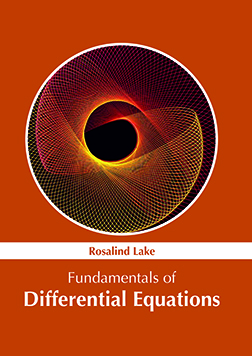BROWSE BY

#### Author

Results 1 - 1 of 1Fundamentals of Differential Equations Author : Rosalind Lake Subject : Chemical Engineering ISBN :9781632386021 The mathematical equations which define the relationship of a function with its derivatives are known as differential equations. The varied types of differential equations include ordinary, partial, non-linear Read More
Results 1 - 1 of 1